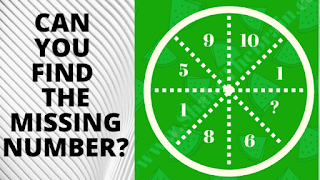This Puzzle Video contains logical reasoning puzzles that will test your brain. In these maths logic puzzles, you will be shown some numbers in the Circle. These numbers follow certain mathematical or logical patterns. Your challenge in these puzzles is to crack the code and find this hidden pattern that relates to the given numbers. Once you are able to crack the code, find the value of the missing number which will replace the question mark.
There are